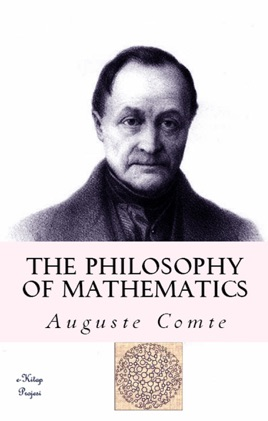• \$4.99

## Publisher Description

In The philosophy of mathematics, mathematics employee classification efforts to understand the philosophy is the branch.
The main question is related to the source of the object that is the subject of mathematics and mathematics. In particular examine the characteris-tics of a true proposition:

• What are the sources of mathematical subject matter?
• What is about the meaning of a mathematical object?
• What is the nature of a mathematical proposition?
• What is the relationship between logic and mathematics?
• What is the role of mathematics hermeneutic?
• Mathematics played a role in the investigation which type
• What is the subject of mathematical investigations?
• What is the human traits behind mathematics?
• What is mathematical beauty?
• What is the nature and source of mathematical truth?
•What is the relationship between mathematics and abstract material universe with the world?

Another important issue is the reality of a mathematical the-ory. Mathematics (from the Natural Sciences as different) experimentally is sought reasons to find real specific mathematical theory can not be tested (see. Epistemology). Luitz that Brouwer 's laid the foundation for intuitionist mathematics of the representatives knew of this view. The logical mathematics is the approach of Bertrand Russell and Gottlob Frege was defended by David Hilbert, formalism is considered the repre-sentative of the current. Traditionalism logician the empiricist's (Rudolf Carnap, A. J. Ayer, Carl Hempel) were represented by one of the key issues in the philosophy of mathematics is also important to regard the certainty problem. Austrian logician Kurt Gödel's also work Mathema-tics and mathematics.